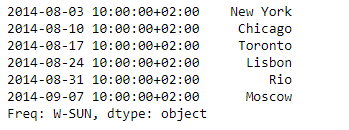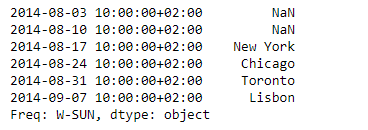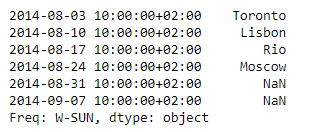Related Articles

# Python | Pandas Series.shift()

• Last Updated : 30 Oct, 2019

Pandas series is a One-dimensional ndarray with axis labels. The labels need not be unique but must be a hashable type. The object supports both integer- and label-based indexing and provides a host of methods for performing operations involving the index.

Pandas` Series.shift()` function shift index by desired number of periods with an optional time freq. When freq is not passed, shift the index without realigning the data.

Syntax: Series.shift(periods=1, freq=None, axis=0, fill_value=None)

Parameter :
periods : Number of periods to shift. Can be positive or negative.
freq : Offset to use from the tseries module or time rule (e.g. ‘EOM’)
axis : Shift direction.
fill_value : The scalar value to use for newly introduced missing values

Returns : Copy of input object, shifted.

Example #1: Use `Series.shift()` function to shift the data of the given Series object by 2 periods.

 `# importing pandas as pd``import` `pandas as pd`` ` `# Creating the Series``sr ``=` `pd.Series([``'New York'``, ``'Chicago'``, ``'Toronto'``, ``'Lisbon'``, ``'Rio'``, ``'Moscow'``])`` ` `# Create the Datetime Index``didx ``=` `pd.DatetimeIndex(start ``=``'2014-08-01 10:00'``, freq ``=``'W'``, ``                     ``periods ``=` `6``, tz ``=` `'Europe/Berlin'``) `` ` `# set the index``sr.index ``=` `didx`` ` `# Print the series``print``(sr)`

Output :Now we will use `Series.shift()` function to shift the data in the given series object by 2 periods.

 `# shift by 2 periods``sr.shift(periods ``=` `2``)`

Output :As we can see in the output, the `Series.shift()` function has successfully shifted the data over the index. Notice the data corresponding to the last two indexes has been dropped.

Example #2: Use `Series.shift()` function to shift the data of the given Series object by -2 periods.

 `# importing pandas as pd``import` `pandas as pd`` ` `# Creating the Series``sr ``=` `pd.Series([``'New York'``, ``'Chicago'``, ``'Toronto'``, ``'Lisbon'``, ``'Rio'``, ``'Moscow'``])`` ` `# Create the Datetime Index``didx ``=` `pd.DatetimeIndex(start ``=``'2014-08-01 10:00'``, freq ``=``'W'``, ``                     ``periods ``=` `6``, tz ``=` `'Europe/Berlin'``) `` ` `# set the index``sr.index ``=` `didx`` ` `# Print the series``print``(sr)`

Output :Now we will use `Series.shift()` function to shift the data in the given series object by -2 periods.

 `# shift by -2 periods``sr.shift(periods ``=` `-``2``)`

Output :As we can see in the output, the `Series.shift()` function has successfully shifted the data over the index. Notice the data of the first two indexes has been dropped.

Attention geek! Strengthen your foundations with the Python Programming Foundation Course and learn the basics.

To begin with, your interview preparations Enhance your Data Structures concepts with the Python DS Course. And to begin with your Machine Learning Journey, join the Machine Learning – Basic Level Course

My Personal Notes arrow_drop_up